# Color Quantization in R

In this post, we’ll look at a simple method to identify segments of an image based on RGB color values. The segmentation technique we’ll consider is called color quantization. Not surprisingly, this topic lends itself naturally to visualization and R makes it easy to render some really cool graphics for the color quantization problem.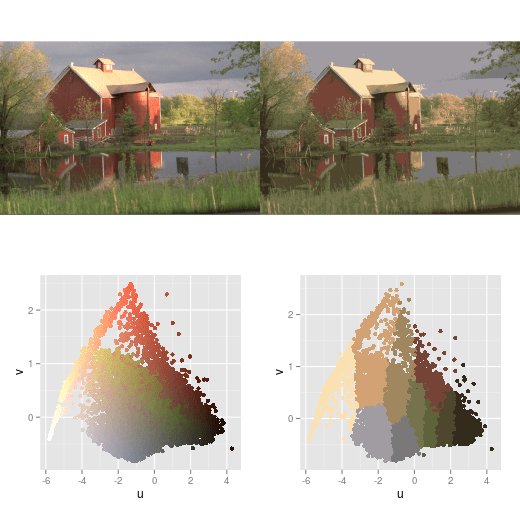The code presented in detail below is packaged concisely in this github gist:

By sourcing this script in R, all the required images will be fetched and some demo visualizations will be rendered.

# Color Quantization

Digital color images can be represented using the RGB color model. In a digital RGB image, each pixel is associated with a triple of 3 channel values red, green, and blue. For a given pixel in the image, each channel has an intensity value (e.g. an integer in the range from 0 to 255 for an 8-bit color representation or a floating point number in the range from 0 to 1). To render a pixel in a particular image, the intensity values of three RGB channels are combined to yield a specific color value. This RGB illumination image from Wikipedia give some idea of how the three RGB channels can combine to form new colors:The goal of image segmentation, is to take a digital image and partition it into simpler regions. By breaking an image into simpler regions, it often becomes easier to identify interesting superstructure in an image such as edges of objects. For example, here’s a possible segmentation of the Wikipedia RGB illumination image into 8 segments: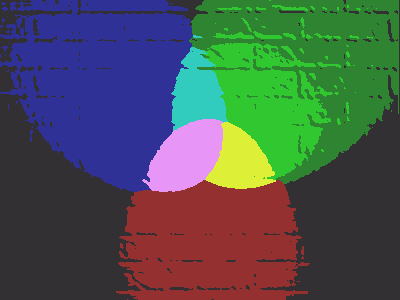This segmentation picks out all of the solid color regions in the original image (excluding the white center) and discards much of the finer details of the image.

There are many approaches to segmenting an image but here we’ll just consider a fairly simple one using K-means. The k-means algorithm attempts to partition a data set into k clusters. Our data set will be the RBG channel values for each pixel in a given image and we’ll choose k to coincide with the number of partitions we’d like to extract from the region. By clustering over the RGB channel values, we’ll tend to get clusters whose RGB channel values are relatively “close” in terms of Euclidean distance. If the choice of k is a good one, the color values of the pixels within a cluster will be very close to each other and the color values of pixels within two different clusters will be fairly distinct.

# Implementing Color Segmentation in R

This beautiful image of a mandrill is famous in image processing (it’s also in the public domain like all images in this post).To load this PNG image into R, we’ll use the PNG package:

library("png")
if(!file.exists("mandrill.png")){
destfile="mandrill.png")
}

# load the PNG into an RGB image object

# This mandrill is 512 x 512 x 3 array
dim(mandrill)
##  512 512   3

In R, an RGB image is represented as an n by m by 3 array. The last dimension of this array is the channel (1 for red, 2 for green, 3 for blue). Here’s what the three RGB channels of the image look like: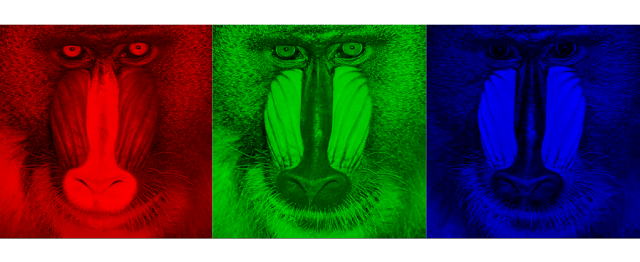Here are some ways to view image data directly from within R:

library("grid")
library("gridExtra")

### EX 1: show the full RGB image
grid.raster(mandrill)

### EX 2: show the B channel in gray scale representing pixel intensity
grid.raster(mandrill[,,3])

### EX 3: show the 3 channels in separate images
# copy the image three times
mandrill.R = mandrill
mandrill.G = mandrill
mandrill.B = mandrill

# zero out the non-contributing channels for each image copy
mandrill.R[,,2:3] = 0
mandrill.G[,,1]=0
mandrill.G[,,3]=0
mandrill.B[,,1:2]=0

# build the image grid
img1 = rasterGrob(mandrill.R)
img2 = rasterGrob(mandrill.G)
img3 = rasterGrob(mandrill.B)
grid.arrange(img1, img2, img3, nrow=1)

Now let’s segment this image. First, we need to reshape the array into a data frame with one row for each pixel and three columns for the RGB channels:

# reshape image into a data frame
df = data.frame(
red = matrix(mandrill[,,1], ncol=1),
green = matrix(mandrill[,,2], ncol=1),
blue = matrix(mandrill[,,3], ncol=1)
)

Now, we apply k-means to our data frame. We’ll choose k=4 to break the image into 4 color regions.

### compute the k-means clustering
K = kmeans(df,4)
df$label = K$cluster

### Replace the color of each pixel in the image with the mean
### R,G, and B values of the cluster in which the pixel resides:

# get the coloring
colors = data.frame(
label = 1:nrow(K$centers), R = K$centers[,"red"],
G = K$centers[,"green"], B = K$centers[,"blue"]
)

# merge color codes on to df
# IMPORTANT: we must maintain the original order of the df after the merge!
df$order = 1:nrow(df) df = merge(df, colors) df = df[order(df$order),]
df$order = NULL Finally, we have to reshape our data frame back into an image: # get mean color channel values for each row of the df. R = matrix(df$R, nrow=dim(mandrill))
G = matrix(df$G, nrow=dim(mandrill)) B = matrix(df$B, nrow=dim(mandrill))

# reconstitute the segmented image in the same shape as the input image
mandrill.segmented = array(dim=dim(mandrill))
mandrill.segmented[,,1] = R
mandrill.segmented[,,2] = G
mandrill.segmented[,,3] = B

# View the result
grid.raster(mandrill.segmented)

Here is our segmented image: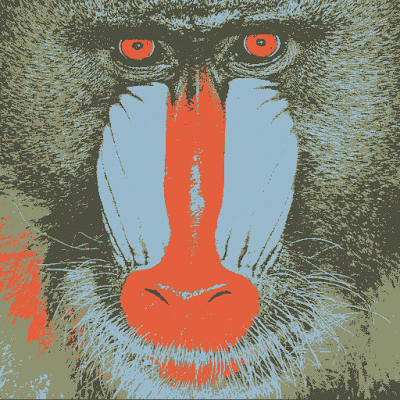# Color Space Plots in Two and Three Dimensions

Color space is the three dimensional space formed by the three RGB channels. We can get a better understanding of color quantization by visualizing our images in color space. Here are animated 3d plots of the color space for the mandrill and the segmented mandrill: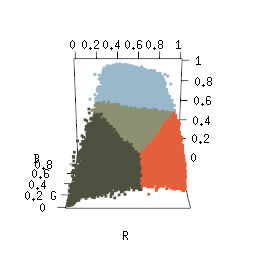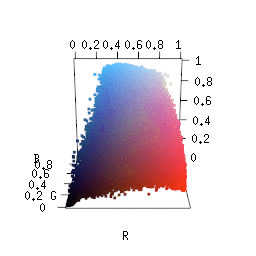These animations were generated with the help of the rgl package:

library("rgl")
# color space plot of mandrill
open3d()
plot3d(df$red, df$green, df$blue, col=rgb(df$red, df$green, df$blue),
xlab="R", ylab="G", zlab="B",
size=3, box=FALSE, axes=TRUE)
play3d( spin3d(axis=c(1,1,1), rpm=3), duration = 10 )

# color space plot of segmented mandrill
open3d()
plot3d(df$red, df$green, df$blue, col=rgb(df$R, df$G, df$B),
xlab="R", ylab="G", zlab="B",
size=3, box=FALSE)
play3d( spin3d(axis=c(1,1,1), rpm=3), duration = 10 )

# Use
# movie3d( spin3d(axis=c(1,1,1), rpm=3), duration = 10 )
# instead of play3d to generate GIFs (requires imagemagick).

To visualize color space in two dimensions, we can use principle components analysis. Principle components transforms the original RGB coordinate system into a new coordinate system UVW. In this system, the U coordinate captures as much of the variance in the original data as possible and the V coordinate captures as much of the variance as possible after factoring out U. So after performing PCA, most of the variation in the data should be visible by plotting in the UV plane. Here is the color space projection for the mandrill: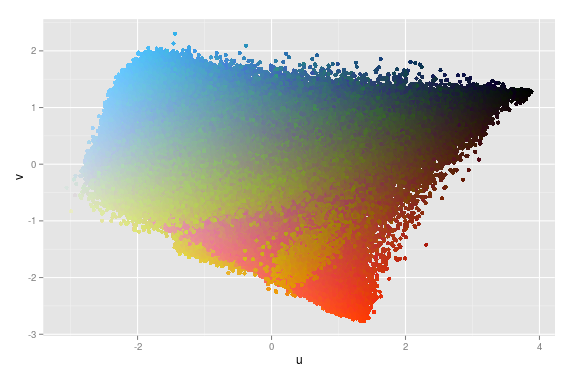and for the segmented mandrill: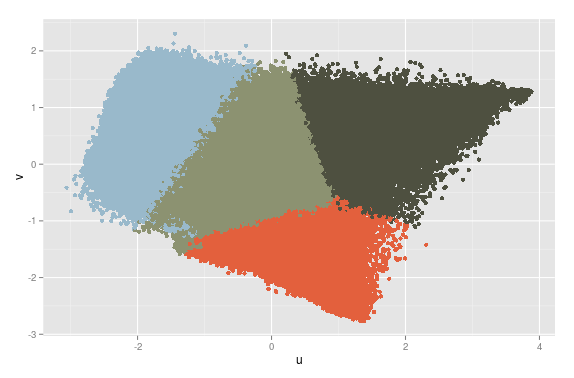Here is the code to generate these projections:

require("ggplot2")

# perform PCA on the mandril data and add the uv coordinates to the dataframe
PCA = prcomp(df[,c("red","green","blue")], center=TRUE, scale=TRUE)
df$u = PCA$x[,1]
df$v = PCA$x[,2]

# Inspect the PCA
# most of the cumulative proportion of variance in PC2 should be close to 1.
summary(PCA)

#Importance of components:
#                          PC1    PC2     PC3
#Standard deviation     1.3903 0.9536 0.39695
#Proportion of Variance 0.6443 0.3031 0.05252
#Cumulative Proportion  0.6443 0.9475 1.00000

# mandrill
ggplot(df, aes(x=u, y=v, col=rgb(red,green,blue))) +
geom_point(size=2) + scale_color_identity()

# segmented mandrill
ggplot(df, aes(x=u, y=v, col=rgb(R,G,B))) +
geom_point(size=2) + scale_color_identity()